# How to Count Cells More Than Certain number of Characters in Excel

This post will guide you how to count the number of cells that more than a certain number of characters in a given range cells using a formula in Excel 2013/2016.How do I count cells with length that greater than a specific number (10) in Excel.

## Count Cells that Contain More Than a Certain Number 10

Assuming that you want to count cells that contain more than a certain number of characters in a selected range(A1:A6).In this case, you can use a formula based on the SUMPRODUCT function and the N function.

Enter the following formula in a blank cell, and press Enter key:

=SUMPRODUCT(N(LEN(A1:A6)>10))

Or

= SUMPRODUCT(–(LEN(A1:A6)>10))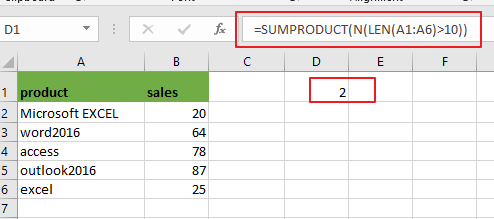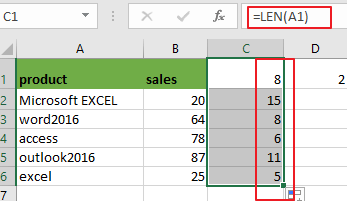Note: A1:A6 is the data range that you want to use. `LEN(A1:A6)>10` is a condition that that data need to be matched.

Now Let’s see how this formula works:

The LEN function will calculate the length for the selected range of cells. Since multiple values were passed into the LEN function and it should be return multiple results as an array list like this: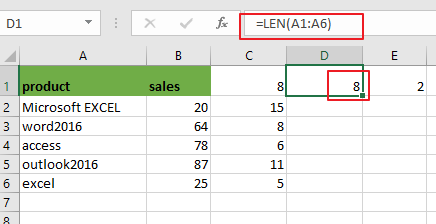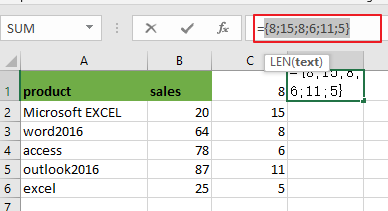={8;15;8;6;11;5}

The LEN function will combine with a logic expression “>10”, and it will return multiple results in an array also like below: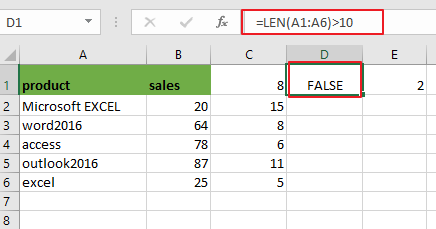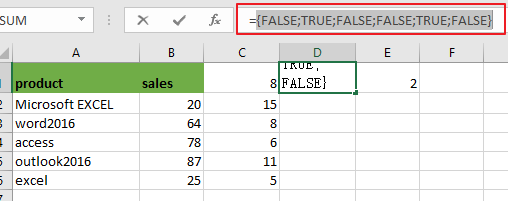={FALSE;TRUE;FALSE;FALSE;TRUE;FALSE}

Then the above array result need to be converted to ones and zeros through the N function or double subtract operators like below:

=–(LEN(A1:A6)>10)

Or

=N(LEN(A1:A6)>10)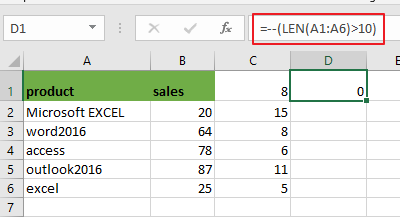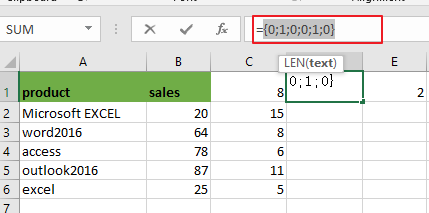Array result is like as below:

={0;1;0;0;1;0}

Last, the above array result contain zeros and ones should be counted by SUMPRODUCT function, it returns 2.

### Related Functions

• Excel SUMPRODUCT function
The Excel SUMPRODUCT function multiplies corresponding components in the given one or more arrays or ranges, and returns the sum of those products.The syntax of the SUMPRODUCT function is as below:= SUMPRODUCT (array1,[array2],…)…
• Excel LEN function
The Excel LEN function returns the length of a text string (the number of characters in a text string).The syntax of the LEN function is as below:= LEN(text)…
• Excel N function
The Excel LEN function returns the length of a text string (the number of characters in a text string).The syntax of the LEN function is as below:= LEN(text)…

Related Posts

Check Cell If Contains One of Many with Exclusions

In Microsoft Excel Spreadsheet or google sheets, when cells contain multiple strings, how can we accomplish this task if you want to check whether these cells contain more than one given string and exclude other given strings? In this article, ...

Cash Denomination Calculator

Every country has different cash denominations, so you may need to calculate the number of different denominations based on the total amount. If it is just a small amount of cash, then you can calculate the different cash denominations manually, ...

Calculate Win Loss Tie

Suppose you got a task to calculate the win, loss, and tie totals; what would you do? If you are new to Ms Excel and don't have enough experience with it, then you might do this task manually but let ...

Count Cells that are Case Sensitive

If you are a frequent user of Microsoft Excel, you may have come across situations where you needed to add or count the cells that were case-sensitive. If you have done this task manually, I would like to point out ...

Check Dates in chronological order

Assume you have a date list that has different date formats, as seen in the accompanying picture. In this instance, Excel's Sort function will fail to sort them appropriately. However, you may convert all various date formats to a particular ...

Sidebar